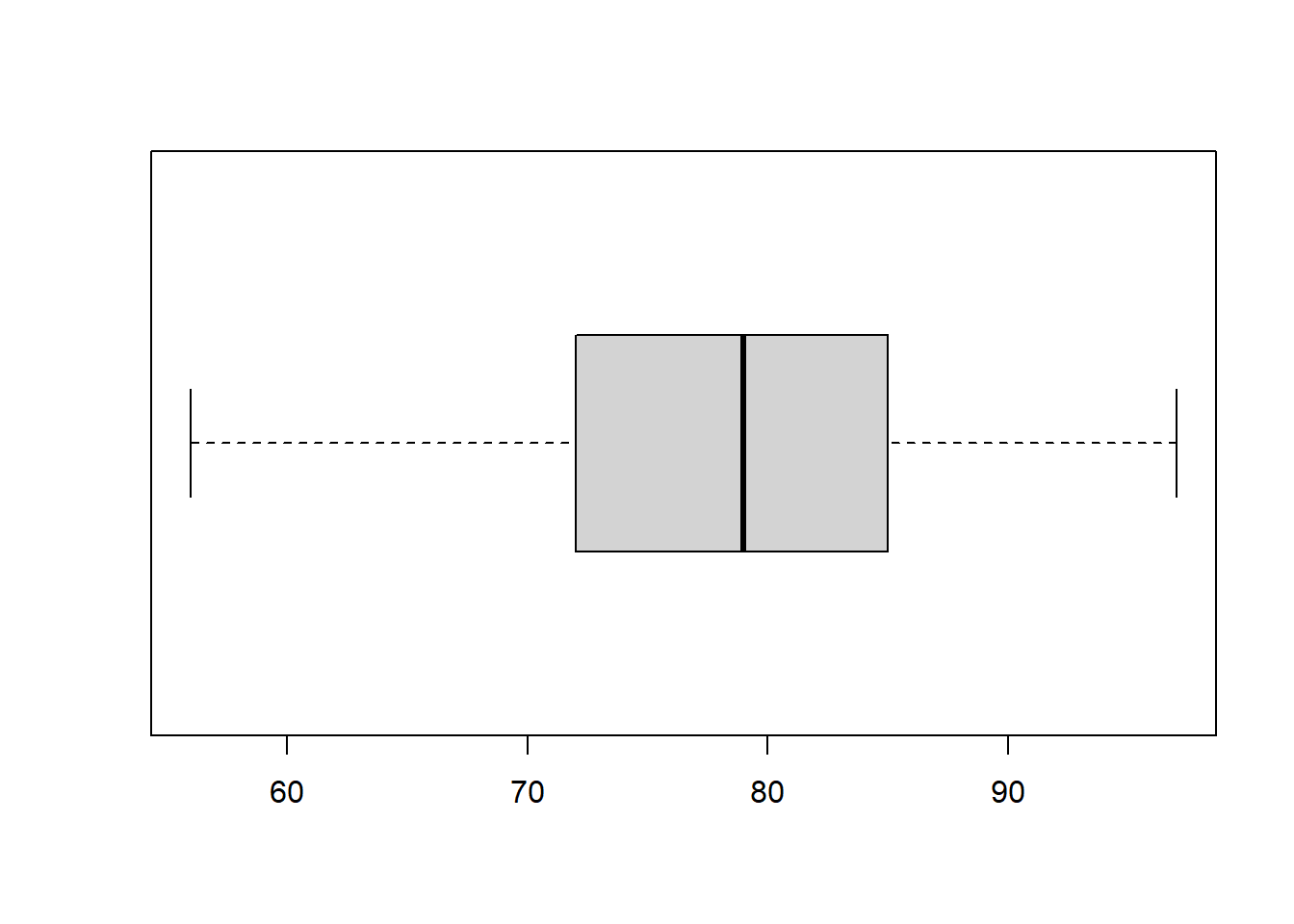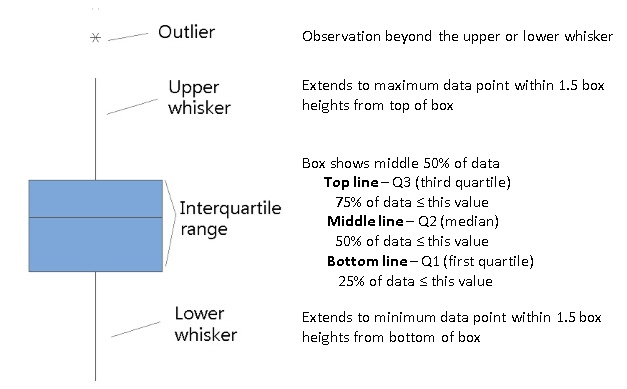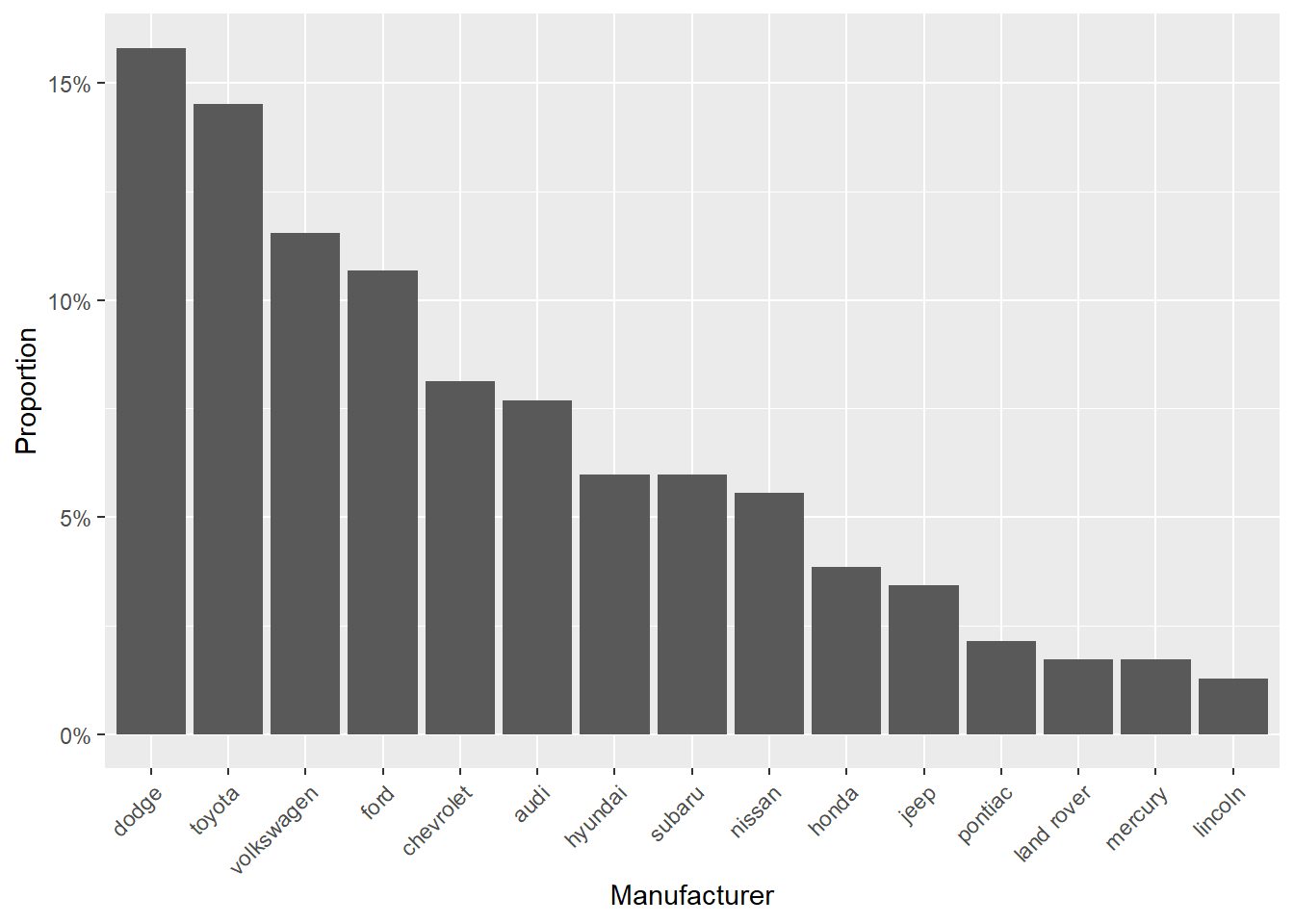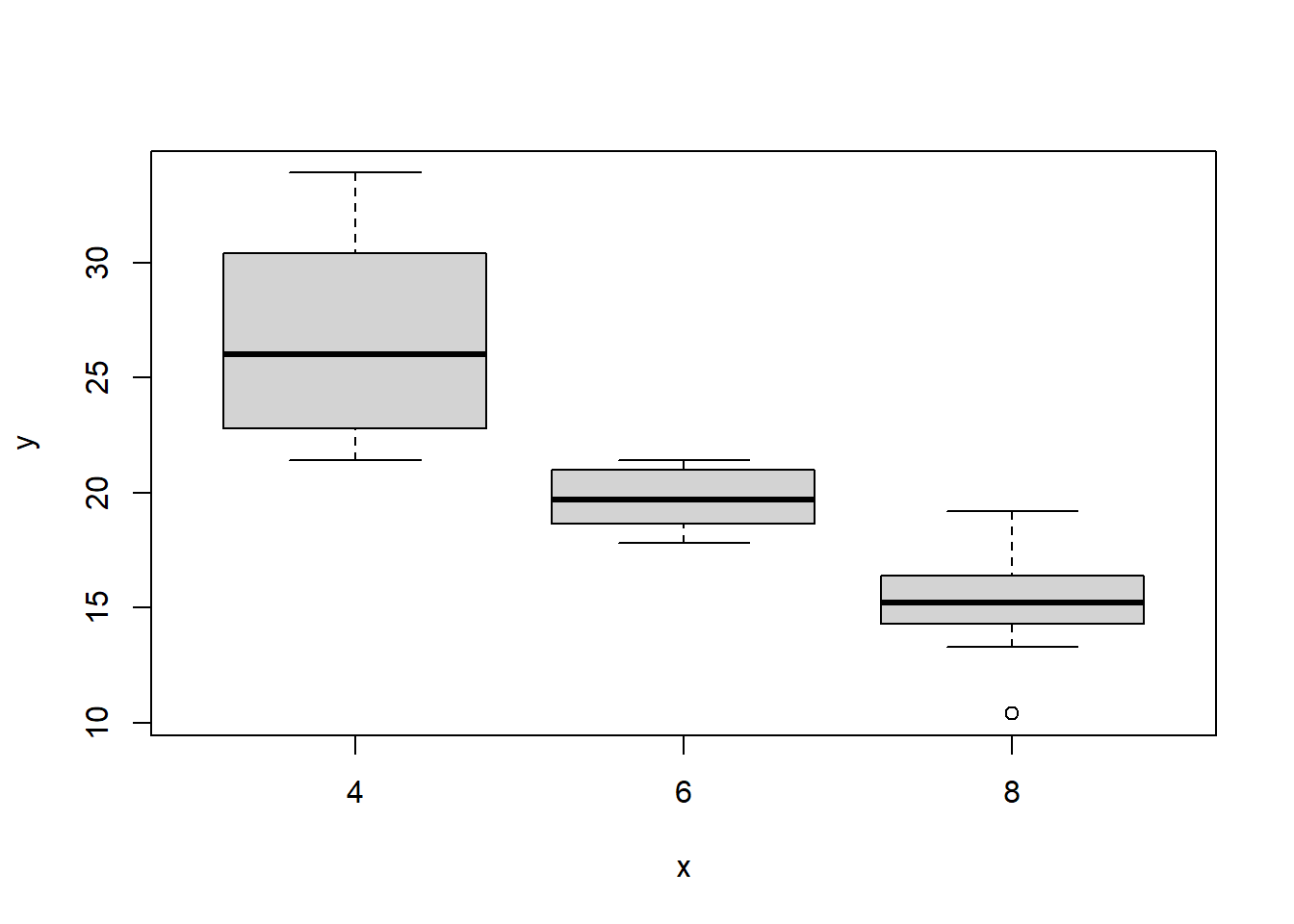# 1 Types of Variables

Variables can be classified:

1. Discrete Variables: variables that assume only a finite number of values. Discrete variables may be further subdivided into:
• Dichotomous variables (takes two possible values): disease status categorized as disease/no disease
• Categorical variables (or nominal variables) (two or more categories): race categorized as non-Hispanic white, Hispanic, black, Asian, other.
• Ordinal variables (there is clear order in the categories unlike categorical variables): level categorized as low, medium, high.
1. Continuous Variables: These are sometimes called quantitative or measurement variables; they can take on any value within a range of plausible values. For example, height, weight, systolic blood pressure, and total serum cholesterol level.

# 2 Descriptive statistics for continuous variables

Daily air quality measurements in New York, May to September 1973. ?airquality.

d <- airquality
head(d)

Temperature (degrees F)

d$Temp ##  67 72 74 62 56 66 65 59 61 69 74 69 66 68 58 64 66 57 68 62 59 73 61 61 57 ##  58 57 67 81 79 76 78 74 67 84 85 79 82 87 90 87 93 92 82 80 79 77 72 65 73 ##  76 77 76 76 76 75 78 73 80 77 83 84 85 81 84 83 83 88 92 92 89 82 73 81 91 ##  80 81 82 84 87 85 74 81 82 86 85 82 86 88 86 83 81 81 81 82 86 85 87 89 90 ##  90 92 86 86 82 80 79 77 79 76 78 78 77 72 75 79 81 86 88 97 94 96 94 91 92 ##  93 93 87 84 80 78 75 73 81 76 77 71 71 78 67 76 68 82 64 71 81 69 63 70 77 ##  75 76 68 Measures of central tendency There are three common measures of central tendency, all of which try to answer the basic question of which value is the most “typical.” These are the mean (average of all observations), median (middle observation), and mode (appears most often). Sample Mean $\bar x = \frac{\sum_{i=1}^n x_i}{n}$ mean(d$Temp)
##  77.88235
median(d$Temp) ##  79 table(d$Temp)
##
## 56 57 58 59 61 62 63 64 65 66 67 68 69 70 71 72 73 74 75 76 77 78 79 80 81 82
##  1  3  2  2  3  2  1  2  2  3  4  4  3  1  3  3  5  4  4  9  7  6  6  5 11  9
## 83 84 85 86 87 88 89 90 91 92 93 94 96 97
##  4  5  5  7  5  3  2  3  2  5  3  2  1  1
getmode <- function(v) {
uniqv <- unique(v)
uniqv[which.max(tabulate(match(v, uniqv)))]
}
getmode(d$Temp) ##  81 Variability The central tendencies give a sense of the most typical values but do not provide with information on the variability of the values. Variability measures provide understanding of how the values are spread out. Range corresponds to maximum value minus the minimum value. min(d$Temp)
##  56
max(d$Temp) ##  97 max(d$Temp)-min(d$Temp) ##  41 range(d$Temp)
##  56 97

Percentiles and quartiles. For any percentage $$k$$%, the $$k$$th percentile is the value $$x$$ such that a percentage $$k$$% of all values are less than it. The $$k$$th percentile of a set of values divides them so that $$k$$% of the values lie below and $$(100-k)$$% of the values lie above.

Percentiles answer questions like this: What is the temperature value $$x$$ such that 90% of temperatures is below it?

Quantiles are the same as percentiles, but are indexed by sample fractions rather than by sample percentages (e.g., 90th percentile or 0.90 quantile).

$P(X < x) = 0.90$The first, second, and third quartiles are the percentiles corresponding to k=25%, k=50%, and k=75%. These three values divide the data into four groups, each with (approximately) a quarter of all observations. Note that the second quartile is equal to the median.

Interquartile range (IQR) corresponds to the difference between the first and third quartiles.# default quantile() percentiles are 0% (minimum), 25%, 50%, 75%, and 100% (maximum)
# provides same output as fivenum()
quantile(d$Temp, na.rm = TRUE) ## 0% 25% 50% 75% 100% ## 56 72 79 85 97 # we can customize quantile() for specific percentiles quantile(d$Temp, probs = seq(from = 0, to = 1, by = .1), na.rm = TRUE)
##   0%  10%  20%  30%  40%  50%  60%  70%  80%  90% 100%
## 56.0 64.2 69.0 74.0 76.8 79.0 81.0 83.0 86.0 90.0 97.0
# we can quickly compute the difference between the 1st and 3rd quantile
IQR(d$Temp) ##  13 An alternative approach is to use the summary() function used to produce min, 1st quantile, median, mean, 3rd quantile, and max summary measures. summary(d$Temp)
##    Min. 1st Qu.  Median    Mean 3rd Qu.    Max.
##   56.00   72.00   79.00   77.88   85.00   97.00

Variance. Although the range provides a crude measure of variability and percentiles/quartiles provide an understanding of divisions of the data, the most common measures to summarize variability are variance and standard deviation.

Sample Mean $\bar x = \frac{\sum_{i=1}^n x_i}{n}$

Sample Variance $s^2 = \frac{\sum_{i=1}^n (x_i-\bar x)^2}{n-1}$

Sample Standard Deviation $s = \sqrt{s^2}$

($$s^2$$ is an unbiased estimator of the population variance $$\sigma^2$$, $$E[s^2]=\sigma^2$$)

# variance
var(d$Temp) ##  89.59133 # standard deviation sd(d$Temp)
##  9.46527

Summaries data frame

We can also get summary statistics for multiple columns at once, using the apply() function.

apply(d, 2, mean, na.rm = TRUE)
##      Ozone    Solar.R       Wind       Temp      Month        Day
##  42.129310 185.931507   9.957516  77.882353   6.993464  15.803922

Summaries on the whole data frame.

summary(d)
##      Ozone           Solar.R           Wind             Temp
##  Min.   :  1.00   Min.   :  7.0   Min.   : 1.700   Min.   :56.00
##  1st Qu.: 18.00   1st Qu.:115.8   1st Qu.: 7.400   1st Qu.:72.00
##  Median : 31.50   Median :205.0   Median : 9.700   Median :79.00
##  Mean   : 42.13   Mean   :185.9   Mean   : 9.958   Mean   :77.88
##  3rd Qu.: 63.25   3rd Qu.:258.8   3rd Qu.:11.500   3rd Qu.:85.00
##  Max.   :168.00   Max.   :334.0   Max.   :20.700   Max.   :97.00
##  NA's   :37       NA's   :7
##      Month            Day
##  Min.   :5.000   Min.   : 1.0
##  1st Qu.:6.000   1st Qu.: 8.0
##  Median :7.000   Median :16.0
##  Mean   :6.993   Mean   :15.8
##  3rd Qu.:8.000   3rd Qu.:23.0
##  Max.   :9.000   Max.   :31.0
## 

Summaries statistics per group

When dealing with grouped data, we will often want to have various summary statistics computed within group (e.g., a table of means and standard deviations). This can be done using the tapply() command. For example, we might want to compute the mean temperatures in each month:

tapply(airquality$Temp, airquality$Month, mean)
##        5        6        7        8        9
## 65.54839 79.10000 83.90323 83.96774 76.90000

Visualization

Histograms display a 1D distribution by dividing into bins and counting the number of observations in each bin. Whereas the previously discussed summary measures - mean, median, standard deviation, skewness - describes only one aspect of a numerical variable, a histogram provides the complete picture by illustrating the center of the distribution, the variability, skewness, and other aspects in one convenient chart.

hist(d$Temp)Boxplots are an alternative way to illustrate the distribution of a variable and is a concise way to illustrate the standard quantiles, shape, and outliers of data. The box itself extends from the first quartile to the third quartile. This means that it contains the middle half of the data. The line inside the box is positioned at the median. The lines (whiskers) coming out either side of the box extend to 1.5 interquartlie ranges (IQRs) from the quartlies. These generally include most of the data outside the box. More distant values, called outliers, are denoted separately by individual points.boxplot(d$Temp)# 3 Descriptive statistics for discrete variables

Fuel economy data from 1999 to 2008 for 38 popular models of cars. ?ggplot2::mpg.

library(ggplot2)
d <- mpg
head(d)

Frequencies and proportions for categorical variables

# counts for manufacturer categories
table(d$manufacturer) ## ## audi chevrolet dodge ford honda hyundai jeep ## 18 19 37 25 9 14 8 ## land rover lincoln mercury nissan pontiac subaru toyota ## 4 3 4 13 5 14 34 ## volkswagen ## 27 # percentages of manufacturer categories table2 <- table(d$manufacturer)
prop.table(table2)
##
##       audi  chevrolet      dodge       ford      honda    hyundai       jeep
## 0.07692308 0.08119658 0.15811966 0.10683761 0.03846154 0.05982906 0.03418803
## land rover    lincoln    mercury     nissan    pontiac     subaru     toyota
## 0.01709402 0.01282051 0.01709402 0.05555556 0.02136752 0.05982906 0.14529915
## volkswagen
## 0.11538462

Visualization

Bar charts are most often used to visualize categorical variables. Here we can assess the count of customers by location:

barplot(table(d$manufacturer))# 4 Pairs of continuous variables Motor trend car road tests. mtcars. ?mtcars head(mtcars) Data frame with 32 observations on 11 variables. • mpg Miles/(US) gallon • cyl Number of cylinders • disp Displacement (cu.in.) • hp Gross horsepower • drat Rear axle ratio • wt Weight (1000 lbs) • qsec 1/4 mile time • vs Engine (0 = V-shaped, 1 = straight) • am Transmission (0 = automatic, 1 = manual) • gear Number of forward gears • carb Number of carburetors Scatterplot plot(x = mtcars$wt, y = mtcars$mpg)We can also get a scatter plot matrix to observe several plots at once. # passing multiple variables to plot plot(mtcars[, 4:6])# 5 Continuous and categorical variables # if x is a factor it will produce a box plot plot(as.factor(mtcars$cyl), mtcars\$mpg)# boxplot of mpg by cyl
boxplot(mpg ~ cyl, data = mtcars)# 6 Exercise

Carry out a descriptive analysis of variable the Ozone in the airquality dataset.